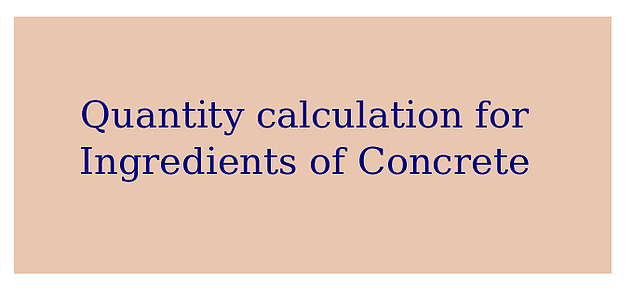+91 80 2574 3350
info@nischinth.com

# Quantity calculation for ingredients of concrete

## Quantity calculation for ingredients of concreteHow to calculate the required quantity of ingredients for 1m³ concrete of grade M20 (Volume batch)?

Given data:

Mix ratio for M20 is 1:1.5:3 (Cement: Fine aggregate: Coarse aggregate)

Solution:

Volume of compacted wet concrete = 1m³

For 1m³ of compacted wet concrete we have to add extra 53% of dry ingredients

So total volume of dry ingredients = 1.53m³

1.Cement

Cement = (1 / (1+1.5+3)) * 1.53               = 0.278m³

2.Fine Aggregate (Sand)

Sand    = (1.5 / (1+1.5+3)) * 1.53             = 0.417m³

3.Coarse aggregate

Coarse aggregate = (3 / (1+1.5+3)) * 1.53 = 0.834m³

Result:

Required quantity of concrete ingredients is as follows

Cement                = 0.278m³

Fine aggregate      = 0.417m³

Coarse aggregate  = 0.834m³

Note: Cement will be procured in bags.

Cement = 0.278*30 (For 1m³ of concrete 30 bags of cement required)  = 8.34 bags